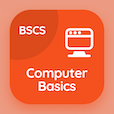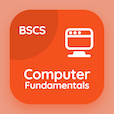Computer Science Courses

Computer Basics Prep Tests

Computer Basics Tests

The Book Hexadecimal Number System Multiple Choice Questions (MCQ Quiz), Hexadecimal Number System quiz answers PDF to learn online courses, computer basics tests. Study Processing Data Multiple Choice Questions and Answers (MCQs), Hexadecimal Number System quiz questions for computer science programs. The App Hexadecimal Number System MCQ e-Book PDF Download: text codes, standard computer ports, microcomputer processor test prep for computer software engineer.

The MCQ: To convert a whole decimal number into a hexadecimal equivalent, one should divide the decimal value by PDF, "Hexadecimal Number System MCQ" App Download (Free) with 2, 8, 10, and 16 choices for computer science programs. Practice hexadecimal number system quiz questions, download Google eBook (Free Sample) for cheapest online computer science degree.

## Computer Basics: Hexadecimal Number System MCQs

MCQ: To convert a whole decimal number into a hexadecimal equivalent, one should divide the decimal value by

A) 2
B) 8
C) 10
D) 16

MCQ: Hexadecimal number system is used as a shorthand language to represent

A) decimal numbers
B) binary numbers
C) octal numbers
D) urinary numbers

MCQ: Numbering system which uses numbers and letters as symbols is termed as

A) decimal
B) binary
C) octal

MCQ: Convert the binary number 1011010 to hexadecimal

A) 5C
B) 5A
C) 5F
D) 5B

### Practice Tests: Computer Basics Exam Prep

The Apps: Computer Basics Quiz App, Computer Fundamentals MCQs App, and Computer Networks MCQ App to download/install for Android & iOS devices. These Apps include complete analytics of real time attempts with interactive assessments. Download Play Store & App Store Apps & Enjoy 100% functionality with subscriptions!